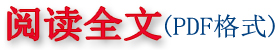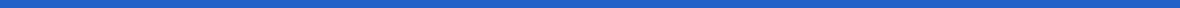阎坤. 数据曲线间断区域的自适应连接方程研究[R]. 西安现代非线性科学应用研究所, 2011 02 20.
 本文框架内容发表在： 阎坤. 数据曲线间断区域的自适应连接方程研究[J]. 地球物理学进展, 2011,26(1):162～171。 YAN Kun. Research on adaptive connection equation in discontinuous area of data curve[J]. Progress in Geophys(in Chinese with abstract in English), 2011, 26(1): 162～171；DOI:10.3969/j.issn.1004-2903.2011.01.018

# 阎 坤 (西安现代非线性科学应用研究所  西安 710061)

 摘  要：通过讨论非线性动力学方程的近似等效解析解及数据曲线间断区域的性质，给出间断区域的自适应连接方程构造形式及其参数确定的预置迭代方法，进而亦给出一般曲线间断区域的映射方程组连接方法，随后给出计算实例，其中包括位错间断区域与回转间断区域的映射方程组及光滑连接曲线。此自适应连接方程，可以作为经典S型曲线方程或Logistic函数的一般扩展形式（扩展型双曲正切函数）予以应用；其中对于缓变数据曲线阶跃间断区域，可自动计算生成连接方程形式。文中分析了非线性方程在经典Newton动力学方程及RLC串联电路电荷方程方面的兼容性；基于自适应连接方程探讨分析了数据曲线或部分连续函数的一种扩展型双曲正切级数近似表示形式、磁性材料磁滞回线方程、粒子统计分布的平均能量方程扩展形式及平均粒子数趋势性微分方程、稳定核素比结合能趋势方程、核素结合能的理论最大值及其相应质子数、势能函数曲线趋势方程（诸如双原子分子势能函数曲线方程）、自然饱和过程方程（诸如钢材料断裂韧性方程）及金属或岩石蠕变过程方程。 关键词：非线性动力学方程，数据曲线间断区域，自适应连接方程，映射连接方程，磁滞回线方程，粒子统计分布方程，比结合能方程，势能函数曲线方程，自然饱和过程方程，蠕变过程方程
 YAN  Kun (Xi’an Modern Nonlinear Science Applying Institute，Xi’an  710061，China)
 Abstract  In this paper, by discussing approximate equivalent analytical solution of nonlinear dynamics equation and some properties in discontinuous area of data curve, a general constructing form of adaptive connection equation and preset iteration method determining parameters in discontinuous area are given. And then, a connecting method of the mapping equations for the discontinuous areas of general curves is also given. Subsequently, computing examples which included the discontinuous areas with dislocation and turn-back are given too. This adaptive connection equation can be applied as a general form of expansion (extended hyperbolic tangent function) of the classical S-curve (sigmoid curve) equation or Logistic function, and for step discontinuous area of slowly varying data curve, its form of the adaptive connection equation can be obtained by automatic calculating. In this paper, compatibility of the nonlinear equation in classical Newton dynamic equation and the charge equation of the RLC series circuit is analyzed. Basing on the form of the adaptive connection equation, an approximate expression of extended hyperbolic tangent series for the data curve or partial continuous functions, equations of magnetic hysteresis loop for magnetic material, extended form of average energy equation (or Einstein-Stern equation) and tendency differential equation of the average particle number for the statistical distributions of the particles, equation of average binding energy per nucleon (or specific binding energy) of stable nuclide, a theoretical maximum of the nuclear binding energy and its corresponding proton number, curvilinear equation of potential energy function (such as curvilinear equation of potential energy function of diatomic molecule, etc), equation of natural saturation process (such as tree growth and physical reaction or chemical reaction process, equation of fracture toughness for steel material, etc) and equation of typical creep process for metal or rock material are explored and analyzed tentatively. Keywords  nonlinear dynamics equation, discontinuous area of data curve, adaptive connection equation, connecting method of the mapping equations, equations of magnetic hysteresis loop, equation of statistical distributions of the particles, equation of average binding energy per nucleon, curvilinear equation of potential energy function, equation of natural saturation process, equation of creep process
 引  言       在自然科学及工程技术的诸多领域，对数据曲线间断区域或等效间断区域予以描述是很重要的研究方向，其尤以确定连接方程构造形式及参数计算方法为关键。    本文通过对非线性方程近似等效解析解及数据曲线在间断区域的讨论，采用趋势分析途径，给出自适应连接方程构造形式及参数的预置迭代计算方法，进而亦给出一般曲线间断区域的映射方程组连接方法，实例计算表明连接效果很好。本文提出了现象演化状态间转变方程，其一般遵循最优光滑曲线路径方程形式的原则；给出并分析了非线性Newton动力学方程、包含二种新的非线性电路元件的RLCNG串联电路方程、数据曲线或连续函数的扩展型双曲正切级数形式及自然演化平衡法则、粒子统计分布的平均能量方程扩展形式及平均粒子数趋势性方程、稳定核素比结合能方程、核素结合能最大值及其质子数、饱和及蠕变过程方程等方面相关内容。    与Bézier函数曲线及相关方法等[2~4]比较，本文方法主要侧重于数据曲线间断区域的自适应连接、或光滑穿过折线区域，其可直接应用到对类如时间序列局部折线及阶跃数据进行自适应光滑处理、曲线间断区域或缠绕区域（等效间断区域）的非线性动力学方程趋势分析描述及简化处理、数据主曲线优化与回归分析融合等方面。相关研究